# Fusion Learning Vignette

#### 2019-03-09

Introduction

Objectives

Data Input

Main Functions

Fusion Learning Algorithm

Unbalanced datasets

Model Selection Criterion

Data Applications

## Introduction

FusionLearn package implements a new learning algorithm to integrate information from different experimental platforms. The algorithm applies the grouped penalization method to select important predictors across multiple datasets. This package can be installed directly from CRAN.

install.packages("FusionLearn",repos = "http://cran.us.r-project.org")

Users can also obtain more information about this package at https://CRAN.R-project.org/package=FusionLearn.

## Objectives

In biological studies, different types of experiments are often conducted to analyze the same biological process, such as disease progression, immune response, etc. High dimensional data from different technologies can be generated to select important biological markers. The conventional approach, such as the penalized likelihood methods can be employed to perform variable selection on high dimensional data using regression model with the biological states as the response variables and the markers measurements as the predictor variables. However, the measurements across different experimental platforms or techniques follow different distributions and models. Measurements generated by different techniques from the same subject can be correlated. The traditional approach is to perform single platform analysis and select a subset of biomarkers based on each of the datasets. However, the selected lists often do not have an agreement across different platforms, and the correlation among the different data sources are not taken into consideration. To address this problem, we develop this R package to integrate correlated information across different platforms and select a consolidated list of biomarkers.

#### High dimensionality

For each platform, a generalized linear model with a linear or logistic link is constructed to model the relationship between the predictors and the responses. For each subject, the response variable measures the biological process of interest, such as the disease status or some quantitative measurement of the disease. The predictors across different platforms are the measurements of thousands of biomarkers. For the same predictor, its regression coefficients from different platforms are grouped into a vector. Then a penalty (LASSO or SCAD) is added to to the $$L_2$$ norm of the estimated vector parameter. By doing so, the estimated predictor effect with small $$L_2$$ norm will be shrunk to zero and deemed to be unimportant predictors. In this case, even if the effect sizes of the same predictor differ from one platform to another, the algorithm will make the decision based on the predictor’s overall $$L_2$$ norm of the effect sizes across all platforms. In high dimension scenario of $$p>n$$, the penalized estimation will enforce the sparsity on the fitted model to avoid overfitting by thresholding the small predictor effect to zero.

#### Heterogeneity

It is often observed that genes perform differently on the different experimental platforms. Such discrepancy could be due to either biological reasons or data inconsistency caused by random variation. Instead of searching for genes with consistent performances across all of the platforms, we select the genes that their overall effect sizes measured by $$L_2$$ norm are great. Based on the overall effect size, the most important genes are selected to produce the candidate list.

#### Dependent vs Independent datasets

In addition, the algorithm offers great flexibility in terms of modeling independent or correlated datasets. If the observations across the platforms are independent, the sample sizes can be different across the datasets. The full likelihood is used to model the independent data. If the samples from different platforms are the same or related, the observations are correlated and the sample sizes will be the same across the platforms. The overall model fitting of all the platforms is evaluated by pseudo-likelihood. The Godambe information matrix of the pseudo-likelihood captures and reflects the covariance structure among different platforms. The new algorithm is able to handle unbalanced data sets across different platforms: 1) the algorithm can deal with the scenario that some predictors are only measured in some but not all platforms by adjusting the penalty size according to the number of platforms in which the predictor is involved; 2) the algorithm allows the sample size to vary from platform to platform if the samples are independent across different platforms.Figure presents an overview of the fusion learning algorithm applied on multiple-platform datasets. The algorithm maximizes the following objective function: $\begin{eqnarray} Q(\beta) = l_I(\beta) - n \sum_{j=1}^p \Omega_{\lambda_n}(||\beta^{(j)}||), \end{eqnarray}$

where $$l_I(\beta)$$ is the integrated pseudo loglikelihood, and $$\Omega_{\lambda_n}$$ represents the penalty function with the tuning parameter $$\lambda_n$$. The penalty function imposes penalty on the $$L_2$$ -norm of the vector of the grouped coefficients ($$\beta^{(j)} = (\beta_{1j}, \beta_{2j}, \cdots, \beta_{mj})^T$$) for each of thepredictors $$M_j$$, $$j=1,\dots,p.$$

Platforms Response $$M_1$$ $$\dots$$ $$M_j$$ $$\dots$$ $$M_p$$
1 $$y_1 \,\text{with}\, g_1(\mu_1) \sim$$ $$x_{11}\beta_{11}$$ $$\dots$$ $$+x_{1j}\beta_{1j}$$ $$\dots$$ $$+x_{1p}\beta_{1p}$$
2 $$y_2 \,\text{with}\,g_2(\mu_2) \sim$$ $$x_{21}\beta_{21}$$ $$\dots$$ $$+x_{2j}\beta_{2j}$$ $$\dots$$ $$+x_{2p}\beta_{2p}$$
$$\cdots$$
m $$y_m\,\text{with}\,g_m(\mu_m) \sim$$ $$x_{m1}\beta_{m1}$$ $$\dots$$ $$+x_{mj}\beta_{mj}$$ $$\dots$$ $$+x_{mp}\beta_{mp}$$

## Data Input

The overall data consists of datasets from multiple platforms. For the $$j$$th platform, the dataset consists of a response vector $$y_j$$ of dimension $$n_j$$ and a predictor matrix $$x_j$$ of dimension $$n_j \times p.$$

Across different platforms, the measurements can be taken from the same samples or related samples, therefore the measurements are dependent across the platforms. If so, the samples across different experiments should be aligned and the sample size remains the same for all experiments.

If the samples from different platforms are unrelated, the observations are independent across the platforms. The sample sizes $$n_j$$ can be different for different platforms.

The predictors across different platforms should be aligned.

We present the simulation of the datasets from four correlated platforms. In each platform, there are 100 samples, and each observation consists of 10 covariates as the candidate variables. The datasets across all platforms are jointly generated as follow.

library(MASS)
library(mvtnorm)
m <- 4
N <- 100
p <- 10
## parameters
rho <- 0.3
Rho <- rho*matrix(1,4,4) + diag(1-rho,4,4)
sigma <- c(2,1,3,2)
Sigma <- Rho * sigma * rep(sigma, each = nrow(Rho))
mu = c(-1,1,0.5,-0.5)

## data information
name_pf =  c("platform 1","platform 2", "platform 3","platform 4")
name_id =  paste("ID", seq(1:N))
name_cov = paste("M", seq(1:p))
x <- array(NA,dim = c(N,m,p))
for(k in 1:p){
x[,,k] <- mvrnorm(N,mu,Sigma)
}
##  "Platform 1"
##         M 1    M 2    M 3    M 4    M 5
## ID 1  1.229 -0.470 -3.709 -3.932 -2.314
## ID 2  1.033 -1.212 -2.453 -1.436 -3.077
## ID 3 -3.316  0.815 -1.624 -2.540 -4.375
## ID 4 -0.964 -1.823 -0.454 -0.240  3.573
## ID 5 -2.844 -2.758 -2.943 -0.743  4.821
##  "Platform 2"
##        M 1   M 2    M 3    M 4    M 5
## ID 1 1.705 1.544 -0.181  1.314 -0.291
## ID 2 1.818 2.620  0.380  1.508  1.036
## ID 3 1.150 2.098  1.553  1.204  0.540
## ID 4 1.830 1.072  2.140  0.823  0.198
## ID 5 1.126 1.608  0.985 -0.156  2.377
##  "Platform 3"
##         M 1    M 2    M 3    M 4    M 5
## ID 1  2.717  1.227  0.469 -0.621  1.586
## ID 2  0.879  4.670  3.152  2.942 -0.964
## ID 3 -3.771  1.326 -2.268 -0.919  4.609
## ID 4  0.563 -0.105 -4.380  2.341  0.557
## ID 5  0.986 -0.094 -1.282 -1.630  3.710
##  "Platform 4"
##         M 1    M 2    M 3    M 4    M 5
## ID 1 -3.475 -2.512 -0.852 -0.862  1.237
## ID 2 -1.573 -0.647  0.508  0.639 -1.028
## ID 3 -2.189  1.394 -1.081 -3.732 -3.739
## ID 4 -1.750  2.265  0.358  1.281  1.099
## ID 5 -1.364 -4.128  3.590 -0.781  1.565

To use the fusion learning functions on these datasets from four platforms, the response vectors and the predictors with the aligned order need to be listed.

x <- list(x1, x2, x3, x4)
y <- list(y1, y2, y3, y4)

## Main Functions

FusionLearn package provides three functions fusionbase, fusionbinary, and fusionmixed, which can be employed to combine continuous, binary, or mixtures of continuous and binary responses from different platforms.

### Fusion Learning Algorithm

#### Continuous Responses

fusionbase is the function applied to the datasets with all continuous response variables. In the function fusionbase, users can set the penalty parameter lambda and choose the penalty functions such as methods = scad or methods = lasso. The function input also include $$N$$, the sample sizes for each platform and $$p$$, the number of the predictors and $$m$$, the number of the platforms. If the sample sizes are all equal, $$N$$ is given as a single value. If the sample sizes are different, $$N$$ is specified as a vector. If some predictors are only measured in some but not all of the platforms, then the datasets do not have complete information and users can specify the option Complete = FALSE . Detailed examples about this type of missing information are shown in Section 4.2.

result <- fusionbase(x, y, lambda = 0.9, N = N ,p = p, m = m, methods = "scad",
Complete = TRUE)
print(result) ## partial estimated parameters
## $beta ## [,1] [,2] [,3] [,4] ## [1,] 2.166467 3.040631 7.785279 8.627343 ## [2,] 3.981514 4.026194 13.773084 11.871934 ## [3,] 3.374323 3.396763 11.606946 9.867747 ## [4,] 4.943008 5.614259 17.270441 15.877448 ## [5,] 4.262251 5.094297 17.604539 16.271033 ## [6,] 0.000000 0.000000 0.000000 0.000000 ## [7,] 0.000000 0.000000 0.000000 0.000000 ## [8,] 0.000000 0.000000 0.000000 0.000000 ## [9,] 0.000000 0.000000 0.000000 0.000000 ## [10,] 0.000000 0.000000 0.000000 0.000000 ## ##$method
##  "scad"
##
## $threshold ##  0.04598454 ## ##$iteration
##  10

The result of the algorithm yields 5 non-zero coefficient vectors and 5 zero coefficient vectors for the 10 predictors. Based on the penalized estimation results, the predictors with non-zero regression coefficients are selected as important predictors. Users can further use the model selection function fusionbase.fit in this package to obtain the value of the pseudo likelihood informaiton criterion for the model.

The algorithm outputs include beta, method, iteration, and threshold. In the output beta, the estimated coefficients are listed. For the example above, the algorithm correctly selects all the five true non-zero coefficients. The figures show the non-zero coefficients and the linear models are well fitted with selected predictors.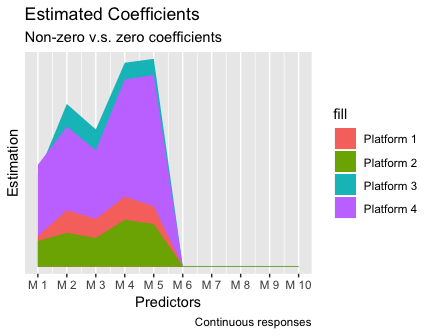Learning example of simulated continuous responses

#### Binary Responses

If the responses from different platforms are all binary variables, users can use the function fusionbinay. Most function inputs are similar to the inputs of the function fusionbase. Users can specify the link functions link = "logit" or link = "probit" for the binary response variables.

result <- fusionbinary(x, y.bin, lambda = 0.15, N = N, p = p, m = m, methods = "scad",
link = "logit")
print(result)
## $beta ## [,1] [,2] [,3] [,4] ## [1,] 0.03466414 0.02843913 0.04444378 0.05617795 ## [2,] 2.05486607 2.01779142 1.13098065 1.59532191 ## [3,] 0.17717603 0.10475392 0.16700634 0.13813861 ## [4,] 2.13573764 2.08503177 2.24617532 1.81413152 ## [5,] 1.24689136 1.11981241 1.84452987 1.91821936 ## [6,] 0.00000000 0.00000000 0.00000000 0.00000000 ## [7,] 0.00000000 0.00000000 0.00000000 0.00000000 ## [8,] 0.00000000 0.00000000 0.00000000 0.00000000 ## [9,] 0.00000000 0.00000000 0.00000000 0.00000000 ## [10,] 0.00000000 0.00000000 0.00000000 0.00000000 ## ##$method
##  "scad"
##
## $link_fn ##  "logit" ## ##$threshold
##  0.09549591
##
## $iteration ##  12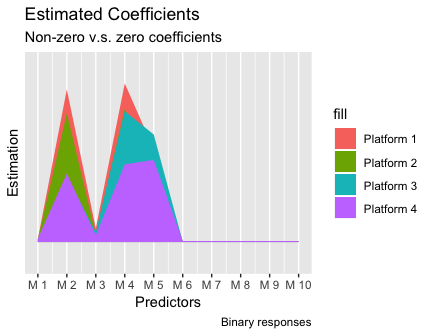Learning example of simulated binary responses ## ## FALSE TRUE ## FALSE 5 0 ## TRUE 0 5 #### Mixed-type Responses If the responses across multiple platforms contain both binary and continuous types, users can use the function fusionmixed. Besides all the inputs similarly required by fusionbase, the function requires the specification of the numbers of the platforms for each type of reponse (m1: the number of the platforms with continuous responses; m2: the number of platforms with binary responses). The link option is used to specify the link function for the binary response variables. result <- fusionmixed(x, y.mixed, lambda = 0.4, N = N, p = p, m1 = 2, m2 = 2, methods = "scad", link = "logit") print(result) ##$beta
##           [,1]     [,2]      [,3]     [,4]
##  [1,] 2.167506 3.030309 0.8995928 1.217401
##  [2,] 3.981460 4.036748 1.5948099 1.790453
##  [3,] 3.374642 3.424883 1.1906511 1.041814
##  [4,] 4.943319 5.613636 2.5516305 2.036690
##  [5,] 4.261845 5.083497 2.2867149 1.988079
##  [6,] 0.000000 0.000000 0.0000000 0.000000
##  [7,] 0.000000 0.000000 0.0000000 0.000000
##  [8,] 0.000000 0.000000 0.0000000 0.000000
##  [9,] 0.000000 0.000000 0.0000000 0.000000
## [10,] 0.000000 0.000000 0.0000000 0.000000
##
## $method ##  "scad" ## ##$link_fn
##  "logit"
##
## $threshold ##  0.09406964 ## ##$iteration
##  12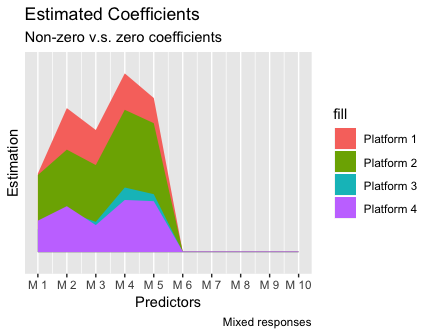Learning example of simulated mixed responses

##
##         FALSE TRUE
##   FALSE     5    0
##   TRUE      0    5

## Unbalanced datasets

In practice, some predictors may not be measured in all of the platforms. Therefore, some of the datasets may not have a complete set of the predictors. The fusion learning package can handle this type of missing predictors. For example, the dataset in platform 2 contains some predictors with all NA values, which means these predictors are not measured in the platform 2. In this case, the group penalization estimation will adjust the penalty with respect to the number of platoforms that predictor is involved in.

##        M 1 M 2    M 3    M 4    M 5
## ID 1 1.705  NA -0.181  1.314 -0.291
## ID 2 1.818  NA  0.380  1.508  1.036
## ID 3 1.150  NA  1.553  1.204  0.540
## ID 4 1.830  NA  2.140  0.823  0.198
## ID 5 1.126  NA  0.985 -0.156  2.377

Since the second predictor is not measured platform 2, the results of corresponding coefficients are NA. For predictors or responses missing at random, various imputation techniques and existing R packages can be employed to impute the missing predictors or responses.

result <- fusionbase(x, y, lambda = 1.3, N = N, p = p, m = m, methods = "scad",
Complete = FALSE)
print(resultbeta) ## [,1] [,2] [,3] [,4] ## [1,] 2.166452 4.563022 7.78528 8.627342 ## [2,] 3.981482 NA 13.77308 11.871933 ## [3,] 3.374330 4.115559 11.60695 9.867745 ## [4,] 4.943011 6.283965 17.27044 15.877448 ## [5,] 4.262262 5.462141 17.60454 16.271033 ## [6,] 0.000000 0.000000 0.00000 0.000000 ## [7,] 0.000000 0.000000 0.00000 0.000000 ## [8,] 0.000000 0.000000 0.00000 0.000000 ## [9,] 0.000000 0.000000 0.00000 0.000000 ## [10,] 0.000000 0.000000 0.00000 0.000000 ## Model Selection Criterion The performance of the variable selection depends on the size of the penalty parameter $$\lambda_n.$$ Larger $$\lambda_n$$ leads to more sparse models. To choose the size of the penalty parameter, users can use model selection criterion. The selection criterion included in FusionLearnis the pseudo-Bayesian information criterion (pseudo-BIC). $\begin{eqnarray}\label{eq:02} \text{pseudo-BIC}(s) = -2l_I(\hat{\beta}_I) + d_s^* \gamma_n, \end{eqnarray}$ with $$-2l_I(\hat{\beta}_I)$$ denoting the pseudo loglikelihood, $$d_s^{*}$$ measuring the model complexity and $$\gamma_n$$ being the penalty on the model complexity. Three functions fusionbase.fit, fusionbinary.fit, and fusionmixed.fit provide the model selection criteria. In the next example, the simulation study is conducted in high dimensional settings. Suppose there are four correlated platforms with mixed-type responses, in which two platforms have continuous responses and two have binary responses. In each platform, we have 500 samples and 1000 predictors. Therefore, the dimension of parameters in each platform is more than the sample size. For a grid of penalty parameter values from $$0.1$$ to $$2$$, function fusionbase.fit can calculate the pseudo-Bayesian information criterion (pseudo-BIC) for each value of the penalty parameter. If the platforms are correlated, the function requires the input depen = "CORR" and the sample sizes across all platforms must be the same and the samples must be aligned across the platforms. The algorithm estimated the Godambe information matrix to capture the dpendence structure across different platforms. There is a multiplicative factor a to the second term in the information criterion . It has a default value of one and users can specify higer values. Increasing the value of a is to impose heavier penalty on the model complexity in the model selection criterion. lambda <- c(0.19, 0.2, 0.3, 0.5, 0.6, 1.75, 2) model <- fusionbase.fit(x, y, lambda, N = 500, p = 1000, m = 4, depen = "CORR")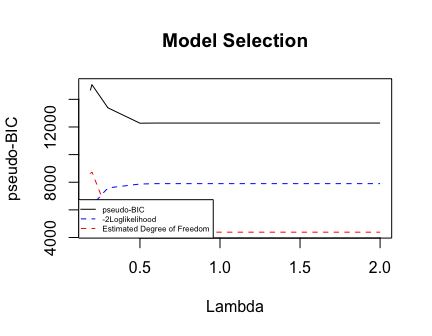Example of model selection process in the fusion learning algorithm print(model) ## lambda BIC -2Loglkh Est_Df Comment ## 1 0.19 14642.59 6030.649 8611.944 . ## 2 0.20 15065.79 6321.654 8744.131 . ## 3 0.30 13386.45 7589.661 5796.789 . ## 4 0.50 12273.36 7869.994 4403.368 The minimum BIC ## 5 0.60 12283.95 7898.730 4385.216 . ## 6 1.75 12283.95 7898.730 4385.216 . ## 7 2.00 12283.95 7898.730 4385.216 . According to the results, when the penalty parameter lambda is $$0.5$$, the selected model has the minimum pseudo-BIC, and the minimum value remains in the range between $$0.19$$ and $$2.00$$. When the penalty parameter is too small, the selected model still has the number of predictors exceeding the number of samples, so that the information matrix can be singular. If the penalty parameter is too large, all predictors’ coefficients will be penalized to zero. Therefore, we can set lambda = 0.5 in the function fusionbase in the next step. result <- fusionbase(x, y, lambda = 0.5, N = 500, p = 1000, m = 4) The function fusionbase provides the results based on the penalty parameter lambda = 0.5. The estimation results indicate that the algorithm correctly identifies the true non-zero and true zero coefficients. ## ## Est zero Est non-zero ## True zero 959 1 ## True non-zero 0 40 ## Data Applications We provide two examples in FusionLearn package. The stock index data is a special application of the fusion learning algorithm on financial data. In this example, the predictors are the 34 stocks, and the three responses are three different market indices. We treat each market index together with the 34 stocks as the dataset from one platform. This data is an example that the financial analysts may be interested in finding the common set of stocks which are influential to all of the three market indices. Since the platforms are correlated, we include the option depen = "CORR" in fusionbase.fit. The plot shows the minimum pseudo-BIC reaches when lambda = 3.34. The selected stocks are listed below. ##analysis of the stock index data #Responses contain indices "VIX","GSPC", and "DJI" y <- list(stockindexVIX[,1],stockindexGSPC[,1],stockindexDJI[,1]) #Predictors include 46 stocks x <- list(stockindexVIX[,2:47],stockindexGSPC[,2:47],stockindexDJI[,2:47]) ##Implementing the model selection algorithm based on the psuedolikelihood ##information criteria model <- fusionbase.fit(x,y,seq(0.03,5,length.out = 10),232,46,3,depen="CORR")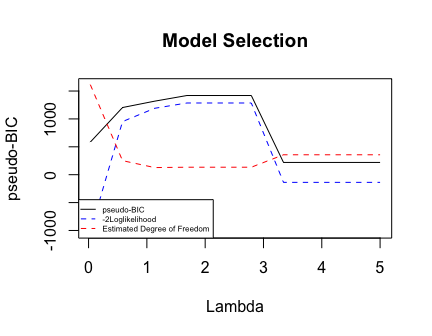Model selection for the function fusionbase.fit print(model) ## lambda BIC -2Loglkh Est_Df Comment ## 1 0.0300000 584.2816 -1030.5640 1614.8456 . ## 2 0.5822222 1204.0856 952.3762 251.7093 . ## 3 1.1344444 1318.3610 1190.6747 127.6863 . ## 4 1.6866667 1419.5304 1285.4696 134.0608 . ## 5 2.2388889 1419.5304 1285.4696 134.0608 . ## 6 2.7911111 1419.5304 1285.4696 134.0608 . ## 7 3.3433333 219.7514 -137.4509 357.2023 The minimum BIC ## 8 3.8955556 219.7514 -137.4509 357.2023 . ## 9 4.4477778 219.7514 -137.4509 357.2023 . ## 10 5.0000000 219.7514 -137.4509 357.2023 . lambda <- model[which.min(model[,2]),1] result <- fusionbase(x,y,lambda,232,46,3) ##Identify the significant predictors for the three indices id <- which(resultbeta[,1]!=0)+1
colnames(stockindexVIX)[id]
##  "DJA" "NYA" "OEX" "RUI"

In the second example, we consider two microarray experiments and both of the experiments were conducted to investigate the estrogen status of breast cancer patients. We treat each experiment as one platform. Since the two experiments are independently conducted, the samples are independent across the two platforms and the sample sizes are different. In particular, one dataset contains 98 observations, and another one includes 286 observations. The predictors in each dataset are 1000 mock-version gene expressions. In the end, the algorithm selects three most important biomarkers to differentiate the estrogen status of the patients.

##Analysis of the gene data
y = list(mockgene1[,2],mockgene2[,2])           ## responses "status"
x = list(mockgene1[,3:502],mockgene2[,3:502])   ## 500 predictors
##Implementing fusion learning algorithm
lambda <- seq(0.1,0.5, length.out = 10)
result <- fusionbinary(x,y,0.3,N=c(98,286),500,2)
id <- which(result\$beta[,1]!=0)+2
genename <- colnames(mockgene1)[id]
print(genename)
##  "200009_at" "205225_at" "209364_at"